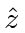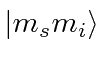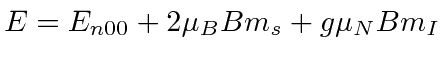## Hyperfine Splitting in a B Field

If we apply a B-field the states will split further. As usual, we choose our coordinates so that the field is indirection. The perturbation then iswhere the magnetic moments from orbital motion, electron spin, and nuclear spin are considered for now. Since we have already specialized to s states, we can drop the orbital term. For fields achievable in the laboratory, we can neglect the nuclear magnetic momentin the perturbation. Then we haveAs an examples of perturbation theory, we will work this problem for weak fields, for strong fields, and also work the general case for intermediate fields. Just as in the Zeeman effect, if one perturbation is much bigger than another, we choose the set of states in which the larger perturbation is diagonal. In this case, the hyperfine splitting is diagonal in states of definitewhile the above perturbation due to the B field is diagonal in states of definite. For a weak field, the hyperfine dominates and we use the states of definite. For a strong field, we use thestates. If the two perturbations are of the same order, we must diagonalize the full perturbation matrix. This calculation will always be correct but more time consuming.

We can estimate the field at which the perturbations are the same size by comparingto. The weak field limit is achieved ifgauss.

* Example: The Hyperfine Splitting in a Weak B Field.*

The result of this is example is quite simple. It has the hyperfine term we computed before and adds a term proportional towhich depends on.

In the strong field limit we use statesand treat the hyperfine interaction as a perturbation. The unperturbed energies of these states are. We kept the small term due to the nuclear moment in the B field without extra effort.

* Example: The Hyperfine Splitting in a Strong B Field.*

The result in this case isFinally, we do the full calculation.

* Example: The Hyperfine Splitting in an Intermediate B Field.*
The general result consists of four energies which depend on the strength of the B field. Two of the energy eigenstates mix in a way that also depends on B. The four energies areandThese should agree with the previous calculations in the two limits: B small, or B large. The figure shows how the eigenenergies depend on B.We can make a more general calculation, in which the interaction of the nuclear magnetic moment is of the same order as the electron. This occurs in muonic hydrogen or positronium. * Example: The Hyperfine Splitting in an Intermediate B Field.*

Jim Branson 2013-04-22